### The Non-Relativistic Equation

Now we will calculate the prediction of the Dirac equation for the non-relativistic coulomb problem, aiming to directly compare to what we have done with the Schrödinger equation for Hydrogen. As for previous Hydrogen solutions, we will set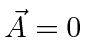but have a scalar potential due to the nucleus. The energy we have been using in our non-relativistic formulation is. We will work with the equation for the large component. Note thatis a function of the coordinates and the momentum operator will differentiate it.Expand the energy term on the left of the equation for the non-relativistic case.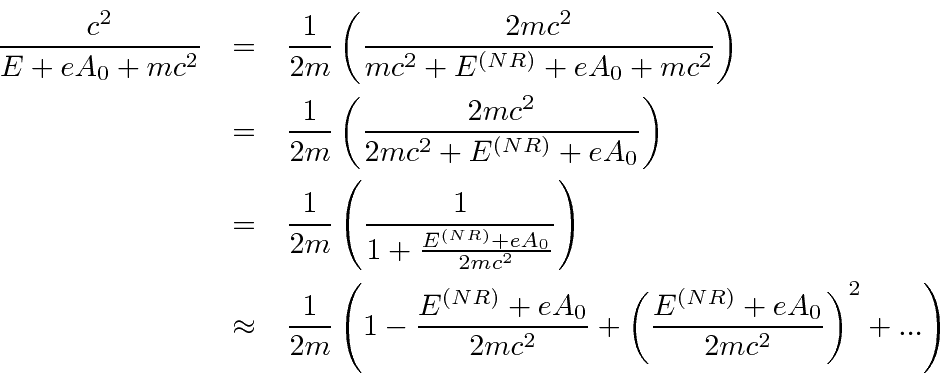We will be attempting to get the correct Schrödinger equation to order, like the one we used to calculate the fine structure in Hydrogen. Since this energy term we are expanding is multiplied in the equation by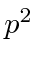, we only need the first two terms in the expansion (order 1 and order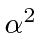).The normalization condition we derive from the Dirac equation isWe've defined, the 2 component wavefunction we will use, in terms ofso that it is properly normalized, at least to order. We can now replacein the equation.This equation is correct, but not exactly what we want for the Schrödinger equation. In particular, we want to isolate the non-relativistic energy on the right of the equation without other operators. We can solve the problem by multiplying both sides of the equation by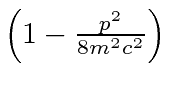.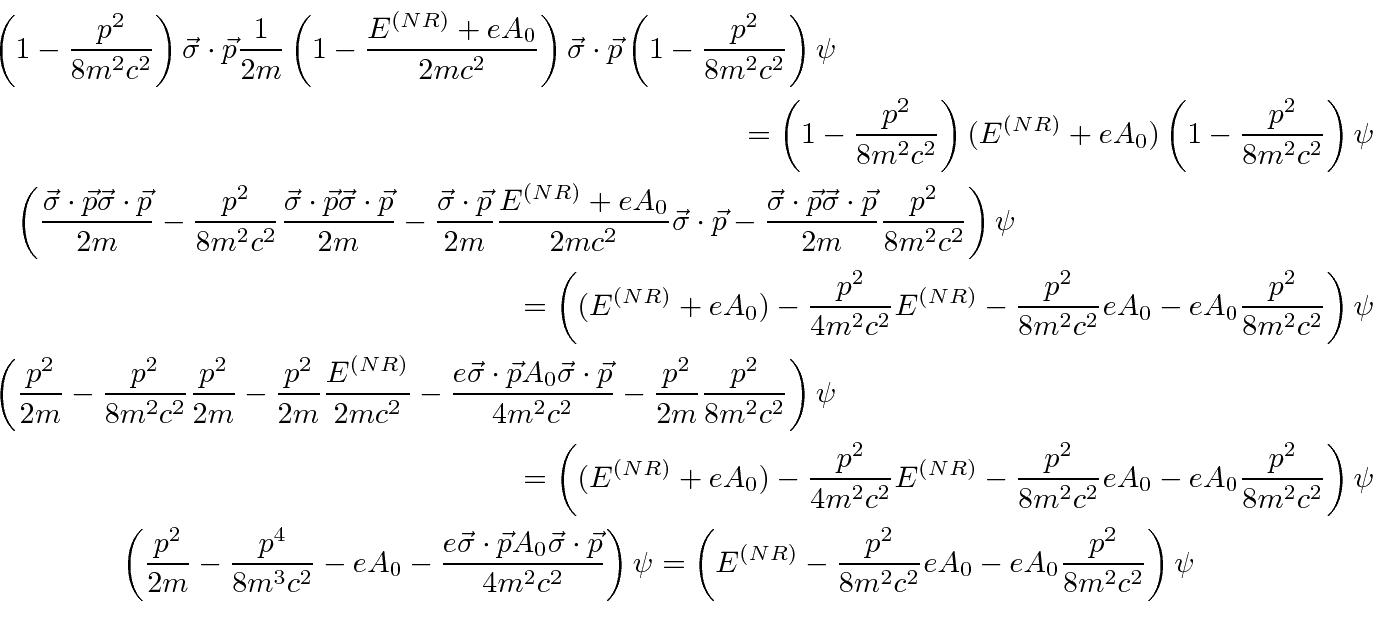We have only kept terms to order. Now we must simplify two of the terms in the equation which contain the momentum operator acting on the field.Plugging this back into the equation, we can cancel several terms.Now we can explicitly put in the potential due to the nucleusin our new units. We identifyas the orbital angular momentum. Note that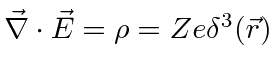. The equation can now be cast in a more familiar form.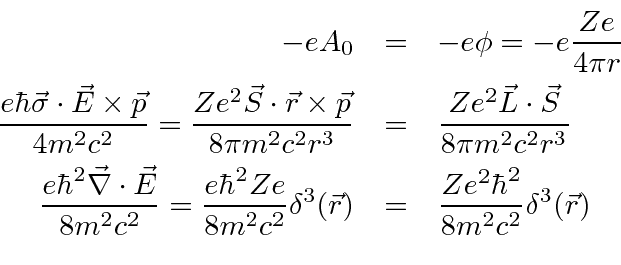This Schrödinger equation'', derived from the Dirac equation, agrees well with the one we used to understand the fine structure of Hydrogen. The first two terms are the kinetic and potential energy terms for the unperturbed Hydrogen Hamiltonian. Note that our units now put ain the denominator here. (Thewill be absorbed into the new formula for.) The third term is the relativistic correction to the kinetic energy. The fourth term is the correct spin-orbit interaction, including the Thomas Precession effect that we did not take the time to understand when we did the NR fine structure. The fifth term is the so called Darwin term which we said would come from the Dirac equation; and now it has.

This was an important test of the Dirac equation and it passed with flying colors. The Dirac equation naturally incorporates relativistic corrections, the interaction with electron spin, and gives an additional correction for s states that is found to be correct experimentally. When the Dirac equation is used to make a quantum field theory of electrons and photons, Quantum ElectroDynamics, we can calculate effects to very high order and compare the calculations with experiment, finding good agreement.

Jim Branson 2013-04-22